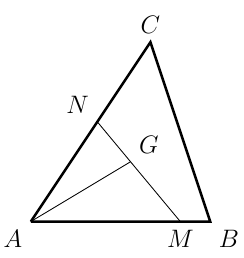back to index | new

Let $z$ be a complex number, and $|z|=1$. Find the maximal value of $u=|z^3-3z+2|$.

Let $x$ be a real number between 0 and 1. Find the maximum value of $x(1-x^4)$.

Let $\alpha, \beta \in (0, \frac{\pi}{2})$. Show that $\alpha + \beta = \frac{\pi}{2}$ if and only if $$\frac{\sin^4 \alpha}{\cos^2 \beta} + \frac{\cos^4\alpha}{\sin^2\beta} = 1$$

Solve in positive integers the equation $x^2y + y^2z +z^2x = 3xyz$

Let $a$ and $b$ be two positive real numbers. Show that if $\frac{1}{a}+\frac{1}{b}=1$. Prove that the following inequality holds for any positive integer $n$: $$(a+b)^n-a^n-b^n\ge 2^{2n}-2^{n+1}$$

If $a\geq b > 1,$ what is the largest possible value of $\log_{a}(a/b) + \log_{b}(b/a)?$

All the numbers $2, 3, 4, 5, 6, 7$ are assigned to the six faces of a cube, one number to each face. For each of the eight vertices of the cube, a product of three numbers is computed, where the three numbers are the numbers assigned to the three faces that include that vertex. What is the greatest possible value of the sum of these eight products?

Let $G$ be the centroid of $\triangle{ABC}$. Points $M$ and $N$ are on side $AB$ and $AC$, respectively such that $\overline{AM} = m\cdot\overline{AB}$ and $\overline{AN} = n\cdot\overline{AC}$ where $m$ and $n$ are two positive real numbers. Find the minimal value of $mn$.A farmer wants to fence in 60,000 square feet of land in a rectangular plot along a straight highway. The fence he plans to use along the highway costs \$2 per foot, while the fence for the other three sides costs \$1 per foot. How much of each type of fence will he have to buy in order to keep expenses to a minimum? What is the minimum expense?

Prove the Maximum Area of a Triangle with Fixed Perimeter is Equilateral

Use the Arithmetic Mean-Geometric Mean Inequality to find the maximum volume of a box made from a 25 by 25 square sheet of cardboard by removing a small square from each corner and folding up the sides to form a lidless box.

Show that for any integer $n\ge 2$: $n! < \Big(\frac{n+1}{2}\Big)^n$

Let $a_1, a_2, \cdots, a_n$ be positive real numbers such that $a_1\cdot a_2\cdots a_n=1$. Show that $$(1+a_1)(1+a_2)\cdots(1+a_n)\ge 2^n$$

Let ${{a}_{2}}, {{a}_{3}}, \cdots, {{a}_{n}}$ be positive real numbers that satisfy ${{a}_{2}}\cdot {{a}_{3}}\cdots {{a}_{n}}=1$ . Prove that $$(a_2+1)^2\cdot (a_3+1)^3\cdots (a_n+1)^n\ge n^n$$

Let $a, b$ be positive real numbers. Prove $$(a+b)\sqrt{\frac{a+b}{2}} \ge a\sqrt{b} + b \sqrt{a}$$.

If $a>1$, then $$\frac{1}{a-1}+\frac{1}{a} + \frac{1}{a+1} > \frac{3}{a}$$ holds.

Let $a, b$ be positive numbers such that $a+b=1$. Show that $$\Big(a+\frac{1}{a}\Big)^2 +\Big(b+\frac{1}{b}\Big)^2\ge \frac{25}{2}$$

Let $a, b, c$ be positive real numbers. Show that $$6a+4b+5c\ge 5\sqrt{ab} + 3\sqrt{bc} + 7\sqrt{ca}$$

Let $a, b, c$ be positive numbers such that $a+b+c=1$. Prove $$\Big(1+\frac{1}{a}\Big)\Big(1+\frac{1}{b}\Big)\Big(1+\frac{1}{c}\Big)\ge 64$$

(Nesbitt's Inequality) Let $a, b, c$ be positive numbers. Show that $$\frac{a}{b+c}+\frac{b}{c+a}+\frac{c}{a+b}\ge\frac{3}{2}$$

Let $x, y, z$ be strictly positive real numbers. Prove that $$\Big(\frac{x}{y}+\frac{z}{\sqrt{xyz}}\Big)^2+\Big(\frac{y}{z}+\frac{x}{\sqrt{xyz}}\Big)^2+\Big(\frac{z}{x}+\frac{y}{\sqrt{xyz}}\Big)^2 \ge 12$$

Let $x, y, z$ be three distinct positive real numbers such that $x+\sqrt{y+\sqrt{z}}=z+\sqrt{y+\sqrt{x}}$. Show that $40xz<1$

Let real numbers $a_1$, $a_2$, $\cdots$, $a_{2016}$ satisfy $9a_i\ge 11a_{i+1}^2$ for $i=1, 2,\cdots, 2015$. Define $a_{2017}=a_1$, find the maximum value of $$P=\displaystyle\prod_{i=1}^{2016}(a_i-a_{i+1}^2)$$

Cyclic quadrilateral $ABCD$ has $AC\perp BD$, $AB + CD = 12$, and $BC + AD = 13$. Find the greatest possible area for $ABCD$.

Let $f(x)=a_0+a_1x+a_2x^2+\cdots +a_nx^n$ be a $n$-degree polynomial and all its coefficients $a_i$ $(0\le i\le n)$ be either $1$ or $-1$. If $f(x)$ has only real roots, what is the maximum value of $n$?